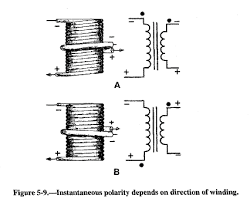# Drawing flux and induced EMF graphs - Faraday's/Lenz's law

Amanda H
I would really appreciate some clarification about how to draw graphs showing changing flux and induced EMF. I understand that there needs to be a 90 degree phase difference as the EMF is a maximum when the flux has the greatest rate of change. However, our exam board says that the EMF can be either leading or lagging by 90 degrees. I don't understand this as surely the as the the EMF = negative gradient of flux then the EMF must be negative when the flux is a positive gradient and vice versa. Please help. This is driving me mad!

Homework Helper
Gold Member
I would really appreciate some clarification about how to draw graphs showing changing flux and induced EMF. I understand that there needs to be a 90 degree phase difference as the EMF is a maximum when the flux has the greatest rate of change. However, our exam board says that the EMF can be either leading or lagging by 90 degrees. I don't understand this as surely the as the the EMF = negative gradient of flux then the EMF must be negative when the flux is a positive gradient and vice versa. Please help. This is driving me mad!
I believe the negative sign in the Lenz's law says that the induced emf "opposes" its cause i.e. change in flux. This means the emf is induced in such a way that the resulting current would try to make up for the change in the original flux.

Look up dot convention in inductors (or transformers). Direction of emf induced between two ends of a coil depends on the sense of the winding.Amanda H
Thank you for helping out. So, what you are saying is that the negative sign in the equation can be ignored when drawing graphs and that the Emf can either lead or lag as this is simply due to the winding...?? This is what I thought but if you see the following link the explanation of the Emf graph describes a positive and negative gradient of the flux...

Homework Helper
Gold Member
Thank you for helping out. So, what you are saying is that the negative sign in the equation can be ignored when drawing graphs and that the Emf can either lead or lag as this is simply due to the winding...?? This is what I thought but if you see the following link the explanation of the Emf graph describes a positive and negative gradient of the flux...